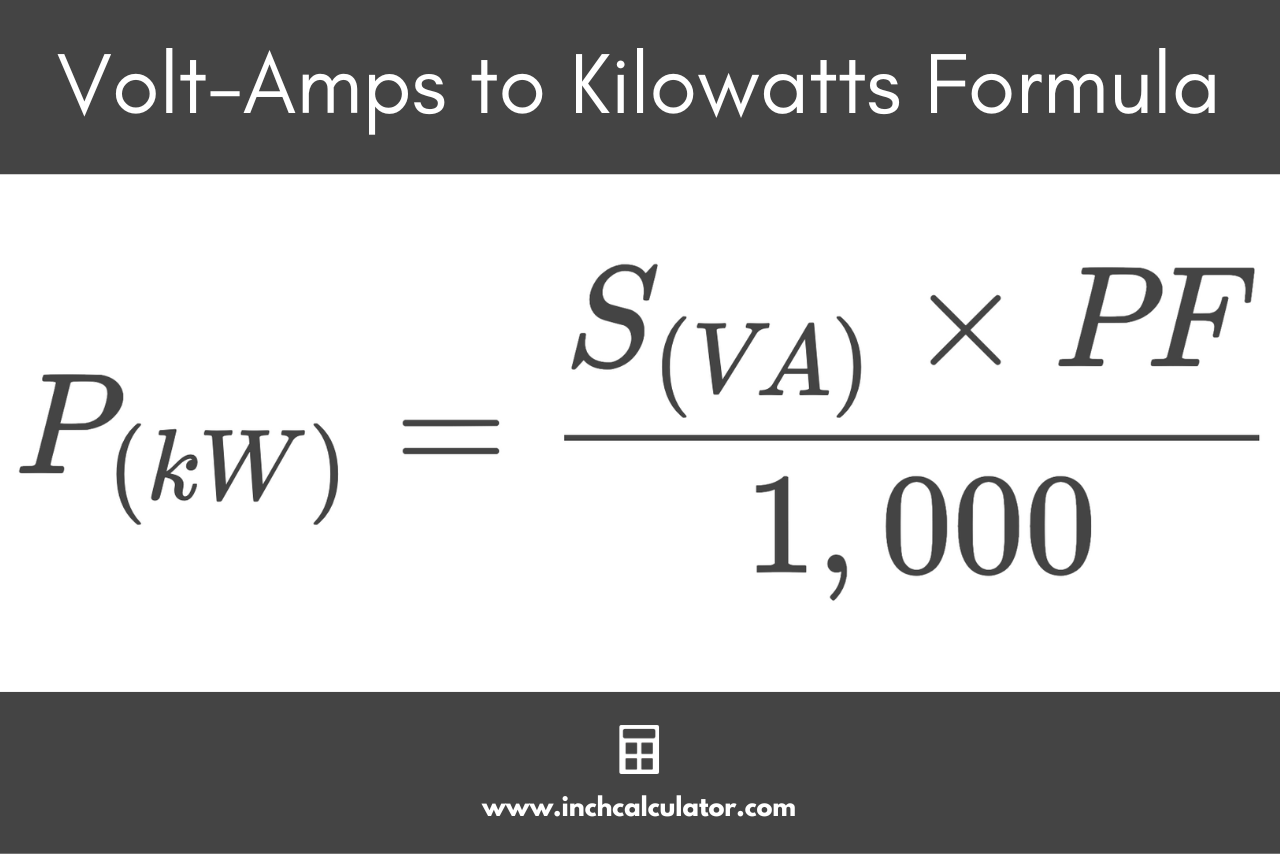# Volt-amps (VA) to Kilowatts (kW) Conversion Calculator

Convert volt-amps (VA) to kilowatts (kW) by entering the power in kW and power factor below.

VA

## Kilowatts Results:

kW
Learn how we calculated this below

## How to Convert Volt-amps to Kilowatts

Both kilowatts and volt-amps measure electrical power. Kilowatts are a measure of real power, while volt-amps are a measure of apparent power.

Electrical power is a measure of the instantaneous rate at which electrical energy is generated at the power station and the total energy delivered to an electrical device connected to the power station.

The generated apparent power is measured in volt-amperes; one VA is equal to the power that would flow when one volt is applied to cause a current flow of one ampere through a device.

The delivered real power is measured in watts (W); one watt equals one joule of energy supplied to a device in one second. In an electrical power system, power is calculated in a higher unit, kilowatts (kW), due to the large electrical loads connected to the power system at any given time. One kW of power is equal to 1,000 watts of power.

The difference between generated apparent power and delivered real power arises due to nonlinear elements of the grid, i.e., the capacitive and inductive elements of the transmission network and connected load. These components have electrostatic and magnetic fields to store energy and require some power loss in terms of reactive power.

The difference between the two is conceptualized by the value of the system power factor, which should ideally be equal to 1. Thus, the total generated apparent power is not always used for real work due to the power factor; converting between them requires a formula.

### VA to kW Conversion Formula

The formula to convert volt-amps to kilowatts is:

P(kW) = S(VA) × PF / 1,000

The real power P in kilowatts is equal to the apparent power S in volt-amps times the power factor PF, divided by 1,000.By reversing this formula, you can also convert kW to VA.

For example, follow this example to convert 45 VA with a power factor of 80% to kilowatts.

P(kW) = 45 VA × 0.8 / 1,000
P(kW) = 36 VA / 1,000
P(kW) = 0.036 kW

## Volt-amps to Kilowatts Conversion Chart

Table showing the apparent power in volt-amps (VA) converted to the real power in kilowatts (kW) using a power factor of 0.8.
Apparent Power (VA) Real Power (kW)
6,250 VA 5 kW
9,375 VA 7.5 kW
12,500 VA 10 kW
18,750 VA 15 kW
25,000 VA 20 kW
31,250 VA 25 kW
37,500 VA 30 kW
50,000 VA 40 kW
62,500 VA 50 kW
75,000 VA 60 kW
93,750 VA 75 kW
125,000 VA 100 kW